Interactive Tutorial about Diffraction Structure solving: Answers part IIIStructure solution Tasks part 1 Answers part 1 Tasks part 2 Answers part 2 Tasks part 3 Answers part 3 Goto Contents The two difference maps had been calculated as: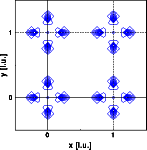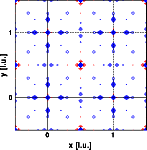The X-ray difference map shows only the oxygen positions, the neutron difference map shows significant differences at the metal positions as well. Why? The metal positions are the correct positions! The phase angles used to calculate the difference Fourier are calculated from the partial structure containing the metal positions only. The X-ray structure factors are dominated by the two metal positions. Most of the phase angles are correct. The neutron scattering lengths of Zirconium and Oxygen are comparable. The scattering length of Titanium is much smaller than the Oxygen scattering length. Omitting the oxygen from the structure introduces many wrong phase angles. The following image illustrates the differences. The most left two images represent the phase angles for the partial structure, left X-ray's right neutron. Each circle represents the phase of a Bragg reflection. Blue circles symbolise a phase of zero, red circles a phase of 180 degrees. The right two images show the corresponding phase angles calculated from the complete structure.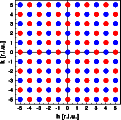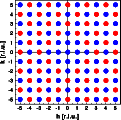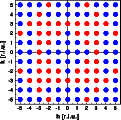In case of X-ray diffraction (left images) only the phase angles of the 10 and 01 reflections calculated from the partial structure deviate from those of the complete structure. In the neutron case, however, many phase angles are wrong. Despite the fact that many neutron phase angles are wrong, the difference Fourier reveals enough information for a complete structure solution. The X-ray example represents a good example for heavy atom methods. The neutron example illustrates that structures can be solved even if a good part of the phase angles is wrong. This is a good point to start an introduction to direct methods. They are, however, beyond the scope of this current tutorial. Look for a guide some time soon. As the last step in our structure determination lets calculate the inverse Fourier. The phase angle have been calculated from the correct atom positions. The left image shows the electron density distribution calculated from the X-ray intensities, the right image the scattering density calculated from the neutron intensities. Note the negative scattering density of the titanium atom.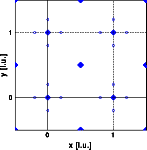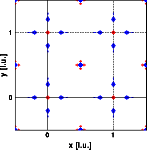The negative scattering density of the titanium and especially the negative scattering density of hydrogen violates the assumption of a non-negative density distribution that is made by direct methods. Again, a good point to start discussing direct methods. © Th. Proffen and R.B. Neder, 2003